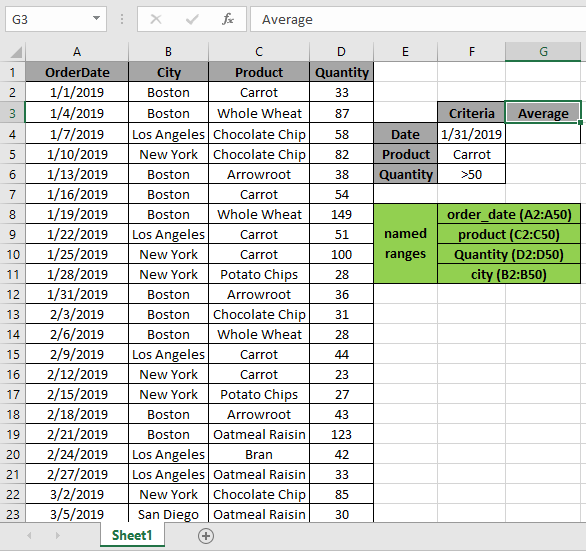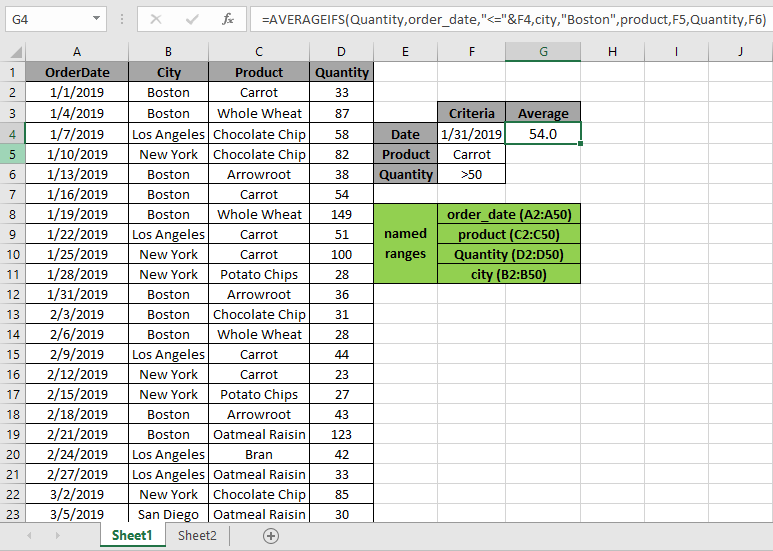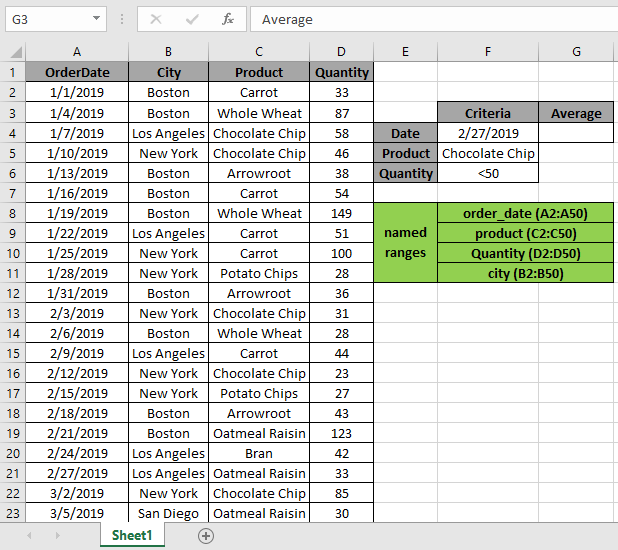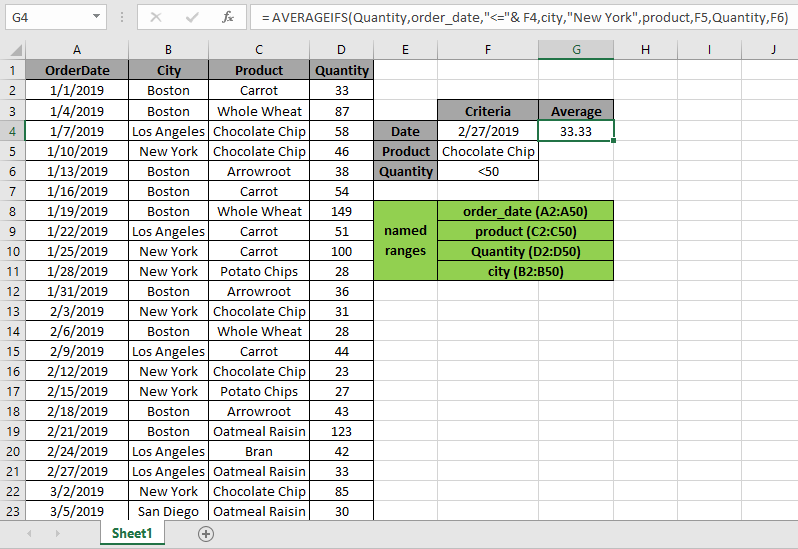# Average with multiple criteria in Excel

In this article, we will learn how to get the Average with multiple criteria in Excel.

Problem?

For Instance, We have a large list of data and we need to find the Average of the price or amount given some criteria. Criteria can be applied over any column of the data.

How to solve the Problem.

Now we will make a formula out of the function. Here we are given the data and we needed to find the AVERAGE of the numbers having some criteria

Generic formula:

= AVERAGEIFS ( Average_range , range1, criteria1, [ range2, criteria2 ], [ range3, criteria3 ], … )
• Average_range : range of values where average needs to be evaluated
• range1 : first range where criteria1 is applied.
• criteria1 : first criteria applied on range1.
• range2 : second range where criteria1 is applied.
• criteria2 : second criteria applied on range2.
• range3 : third range where criteria1 is applied.
• criteria3 : third criteria applied on range3.

Notes: do not provide date directly to the function. Use DATE function or use cell reference for date argument in excel as Excel reads date only with the correct order.

Example:

All of these might be confusing to understand. So, let's test this formula via running it on the example shown below.

Here we have given data from A1:D51. We need to find the AVERAGE of Quantity received as per different criteria.

Conditions are as follows.

1. Date must be before 1/31/2019.
2. City "Boston"
3. Product be defined as "Carrot"
4. Quantity must be greater than 50 ( >50 ).The above 4 stated conditions or criteria needs to be matched. The AVERAGEIFS function can help us extract the average having different criteria.

Use the Formula:

= AVERAGEIFS ( Quantity , order_date , "<=" & F4 , city , "Boston" , product , F5 , Quantity , F6 )

Quantity : named range used for D2:D50 array

Order_date : named range used for A2:A50 array

City : named range used for B2:B50 array

Product: named range used for C2:C50 array

F4 : cell reference used for criteria 1

F5 : cell reference used for criteria 2

F6 : cell reference used for criteria 3

Explanation:

1. order_date , "<=" & F4  first criteria which suggests date before 1/31/2019 in F4.
2. city , "Boston" second criteria where city matches "Boston"
3. product , F5 where product matches Carrot in F5 cell
4. Quantity, must be under criteria in F6 cell i.e greater than 50.

Here the A2 is given as cell reference & Named ranges given as rng ( D2 : D51 ) and order_date ( A2 : A51).As you can see in the above snapshot the AVERAGE of the quantity in January comes out to be 54.00. As there is only one value satisfying all conditions.

Example 2:

Here we have given data from A1:D51. We need to find the AVERAGE of Quantity received as per different criteria.

Conditions are as follows.

1. Date must be before 2/27/2019.
2. City "New York"
3. Product be defined as "Chocolate Chip".
4. Quantity must be less than than 50 ( < 50 ).The above 4 stated conditions or criteria needs to be matched. The AVERAGEIFS function can help us extract the average having different criteria.

Use the Formula:

= AVERAGEIFS ( Quantity , order_date , "<=" & F4 , city , "New York" , product , F5 , Quantity , F6 )

Quantity : named range used for D2:D50 array

Order_date : named range used for A2:A50 array

City : named range used for B2:B50 array

Product: named range used for C2:C50 array

F4 : cell reference used for criteria 1

F5 : cell reference used for criteria 2

F6 : cell reference used for criteria 3

Explanation:

1. order_date , "<=" & F4  first criteria which suggests date before 2/27/2019 in F4 cell.
2. city , "Boston" second criteria where city matches "Boston"
3. product , F5 where product matches Carrot in F5 cell
4. Quantity, must be under criteria in F6 cell i.e less than 50.

Here the A2 is given as cell reference & Named ranges given as rng ( D2 : D51 ) and order_date ( A2 : A51).As you can see in the above snapshot the AVERAGE of the quantity in January comes out to be 33.33... As there is only one value satisfying all conditions.

Here are some observations about the formula usage below.

Notes:

1.  The function returns #VALUE! error if the argument to the function is non-numeric.
2. The function returns #VALUE! error if no value matches the given criteria.
3. The formula accepts operators such as =, < , <= , >= , <= & <>.
4. Do not provide date argument directly to the function. Use DATE function or use cell reference for date argument in excel as Excel reads date only with the correct order.

Hope this article about how to Average with multiple criteria in Excel is explanatory. Find more articles on SUMPRODUCT functions here. Please share your query below in the comment box. We will assist you.

Related Articles

How to use the AVERAGEIFS function in excel

How to use the DAVERAGE Function in Excel

How To Highlight Cells Above and Below Average Value

Ignore zero in the Average of numbers

Calculate Weighted Average

Average Difference between lists

Validation of text entries

Create drop down list in excel with colour

Popular Articles

Edit a dropdown list

Absolute reference in Excel

If with conditional formatting

If with wildcards

Vlookup by date

Join first and last name in excel

Count cells which match either A or B

Convert Inches To Feet and Inches in Excel 2016

50 Excel Shortcut to Increase Your Productivity

Terms and Conditions of use

The applications/code on this site are distributed as is and without warranties or liability. In no event shall the owner of the copyrights, or the authors of the applications/code be liable for any loss of profit, any problems or any damage resulting from the use or evaluation of the applications/code.